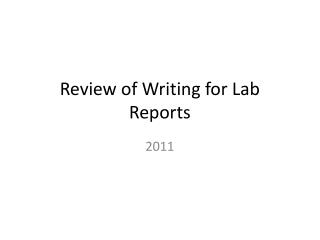DownloadDownload PresentationReview of Writing for Lab Reports

# Review of Writing for Lab Reports

Download Presentation## Review of Writing for Lab Reports

- - - - - - - - - - - - - - - - - - - - - - - - - - - E N D - - - - - - - - - - - - - - - - - - - - - - - - - - -
##### Presentation Transcript

1. Pre-Quiz (part 1) • Which of these sentences is written in the correct format for your Data Analysis section? • A. Use the formula, F=mg, to find the weight of the mass. • B. The formula, F=mg, was used to find the weight of the sled. • C. We used the formula, F=mg, to find the weight of the sled. • Which of these sentences is written in the correct format for your Conclusion section? • A. Our hypothesis was wrong. • B. My hypothesis was wrong. • C. The hypothesis was wrong.

2. Person • What person are your formal solutions written in? • 1st person plural: We use the formula F=ma. • What person are your procedures written in? • 2nd person: (You) place the mass on the sled. • Write an example of a sentence in 3rd person.

3. Voice • Active Voice: The entity doing the action is the subject of the sentence. • I placed the mass on the sled. • Passive Voice: The entity having the action done to it is the subject of the sentence. • The mass was placed on the sled. • Which is more proper? • In an essay, it is more proper to use active voice, but in a lab report, it is sometimes better to use passive voice.

4. Verb tense • When you write your lab report, you will often be reporting on what happened. • It is proper to use past tense for these sections of the report. • What sections do you think will be written ALL in past tense? • Methods: where you will report on how your data was collected • Data Analysis: where you will report on how your data was analyzed

5. DON’T REFER TO YOURSELF IN YOUR REPORT!!!!!! • You have to write in 3rd person. You must write about your procedure and your data analysis without referring to yourself in the third person. • How would you explain how the normal force was found?

6. Let’s try a few examples • Let’s translate a few practice sentences that you may find in your procedure and data sections into the proper person, voice and verb tense. • Weigh the sled by hanging it, vertically, from the spring scale. • The weight of the sled was found by hanging it vertically from the spring scale. • We use the average static friction for each surface to find the coefficient of static friction for that surface. • The average static friction was used to find the coefficient of friction for each surface. • My hypothesis, that the foam rubber would have the highest coefficient of friction, was not correct. • The hypothesis was shown to be incorrect; the foam rubber was not the surface with the highest coefficients of friction.

7. Post-Quiz (part 1) • Which of these sentences is written in the correct format for your Data Analysis section? • A. Use the formula, F=mg, to find the weight of the mass. • B. The formula, F=mg, was used to find the weight of the sled. • C. We used the formula, F=mg, to find the weight of the sled. • Which of these sentences is written in the correct format for your Conclusion section? • A. Our hypothesis was wrong. • B. My hypothesis was wrong. • C. The hypothesis was wrong.